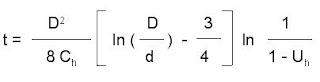### Calculation of PVD's 3 - Kjellman formulawhere:
t = consolidation period (years)
D = diameter of drained soil cylinder (m)
d = equivalent diameter of drain (m)
Ch = horizontal consolidation coefficient (m2/year)
Uh = average horizontal consolidation degree